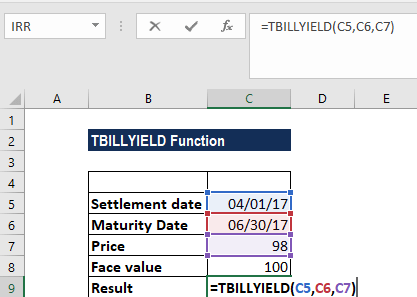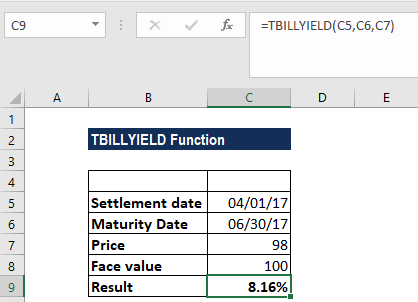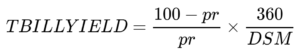# TBILLYIELD Function

Calculates the yield on a Treasury bill

## What is the TBILLYIELD Function?

The TBILLYIELD Function is categorized under Excel FINANCIAL functions. It will calculate the yield on a Treasury bill.

In financial analysis, TBILLYIELD can be useful in calculating the yield on a Treasury bill when we are given the start date, end date, and price.

### Formula

=TBILLYIELD(settlement, maturity, pr)

The TBILLYIELD function uses the following arguments:

1. Settlement (required argument) – This is the settlement date of the Treasury bill. The security’s settlement date is the date after the issue date when the T-bill is traded to the buyer.
2. Maturity (required argument) – This is the T-bill’s maturity date. The maturity date is the date when the T-bill expires.
3. Pr (required argument) – The T-bill’s price per \$100 face value.

### How to use the TBILLYIELD Function in Excel?

As a worksheet function, TBILLYIELD can be entered as part of a formula in a cell of a worksheet. To understand the uses of the function, let us consider an example:

#### Example

Assume we are given the following data:

• Settlement date: 04/01/17
• Maturity date: 06/30/17
• Price: 98
• Face value: 100

Using the TBILLYIELD function, we can calculate the yield. The formula is:We get the results below:### Things to remember about the TBILLYIELD Function

1. #NUM! error – Occurs if either:
1. The settlement date is greater than or equal to the maturity date or the maturity date is more than 1 year after the settlement date
2. The supplied pr argument is less than or equal to 0.
2. #VALUE! error – Occurs if either:
1. Any of the supplied arguments are non-numeric
2. The settlement or the maturity arguments are not valid dates.
3. Settlement and maturity are truncated to integers.
4. TBILLYIELD is calculated as follows:Where:

DSM = number of days from settlement to maturity, excluding any maturity date that is more than one calendar year after the settlement date

1. The settlement and maturity arguments should be supplied to the function as either:
1. References to cells containing dates
2. Dates returned from other functions or formulas.

Thanks for reading CFI’s guide to important Excel functions! By taking the time to learn and master these functions, you’ll significantly speed up your financial modeling and analysis. To learn more, see these additional CFI resources:

• List of Excel Functions
• List of Excel Shortcuts